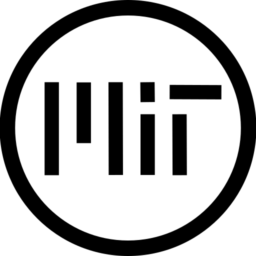# Linear Depth/Depth Fog

‘Linear Depth/DepthFog’ post-processing effect

How to apply:

`1. Create a new Mesh Instance and set the mesh to a Quad`
`2. Set the newly created Quad's size to '2x2'`
`3. Create a new shader material in the 'Material' or 'Surface Material Override' tab`
`4. Create a new shader under the 'Shader' tab`
`5. Copy and paste the shader.`

This shader is a part of my tutorial/learning repository

``````// Linear Depth Shader written by absentSpaghetti
//
// This shader is a part of my tutorial/learning Github repository: https://github.com/absentSpaghetti/Shader-Basics-With-Spaghetti
//
//
// prerequisites:

uniform sampler2D depth_texture : hint_depth_texture, filter_linear_mipmap;
uniform sampler2D screen_texture : hint_screen_texture, filter_linear_mipmap;

//uniform vec4 fog_color : source_color = vec4(0.75);
//uniform float fog_intensity : hint_range(0.0, 1.0, 0.01) = 0.1;

uniform float amount : hint_range(0.01, 1.0, 0.01) = 0.1;

void vertex() {
POSITION = vec4(VERTEX, 1.0);
}

void fragment() {

vec3 screen_color = texture(screen_texture, SCREEN_UV).rgb;

// The depth can be read using this line. The values returned by it are between 0.0 - 1.0 and are non-linear.
// If we plug this directly into the ALBEDO texture, you will notice that everything is white, unless we get very close to it.
// In order to fix this, we need to linearize the value.
float depth = texture(depth_texture, SCREEN_UV).x;
//	ALBEDO = vec3(depth)

// Normalized Device Coordinates ('NDC' for short), are a way of representing point's position on the 2D screen, relative to the width and height of it.
// It ranges from (-1, 1), where the bottom-left corner has a value of (-1, -1) and the top-right corner has a value of (1, 1)
vec3 ndc = vec3((SCREEN_UV * 2.0) - 1.0, depth);
//	ALBEDO = ndc;

// To use the ndc, we need to convert it to the view (camera) space, we can do it by multiplying it by the inverse of the projection matrix.
// Space view gives us values relative to the camera position, which means that the 'z' (forward/backward), will give us the distance
vec4 view = INV_PROJECTION_MATRIX * vec4(ndc, 1.0);
view.xyz /= view.w;
float linear_depth = -view.z; // Resulting value has a range of (-1, 0), which means we have to multiply it by -1 to get positive values
ALBEDO = vec3(linear_depth * amount);

//	// Using this depth, a simple depth fog shader can be written. We just need to mix the screen_color with the fog_color using the depth as a value to interpolate by
//	float fog_factor = clamp(linear_depth * fog_intensity, 0.0, 1.0);
//	vec4 fogged_color = mix(vec4(screen_color, 1.0), fog_color, fog_factor);
//
//	ALBEDO = fogged_color.rgb; // we then apply the fog color and alpha to the albedo and alpha channels
//	ALPHA = fogged_color.a;
}

###### Tags
depth, depth fog, Fog, linear depthThe shader code and all code snippets in this post are under MIT license and can be used freely. Images and videos, and assets depicted in those, do not fall under this license. For more info, see our License terms.

## More from absentSpaghetti

### Fog of war with alpha cut off as white color

Subscribe
Notify of